# The limit of the function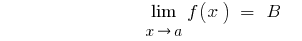Where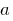is the number or one of the symbols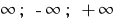Definition: a Numberis called the limit of the function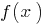at the point(ifthat is sent to), if for any positivethere is a positive number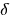that for all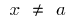that satisfy the inequality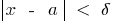, the following inequality is satisfied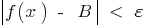## Mathematical notation expressions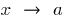and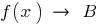The pointis from pointat short distances (less)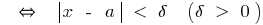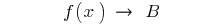The valueson the number line is located at a small distance(less than)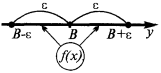## Properties of limit of a function

1. If, then, the only
2. If the functionhas a limit at a, then the limit is the only

3.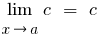where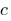is a constant
4. The limit of a constant function is equal to the same constant

5.— continuous at the pointa function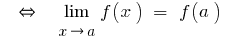(by definition of a continuous function
6. Border nemerenno function when itis equal to the value of the function at the point## Theorems on limits

1.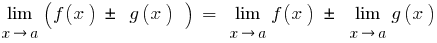2.3.4.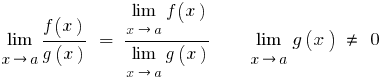## The first significant boundary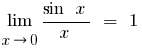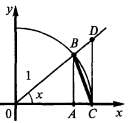Tags:
Chapter:
Versions in other languages:
Share with friends: